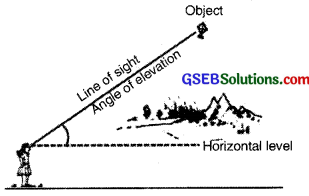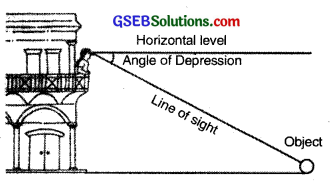# GSEB Class 10 Maths Notes Chapter 9 Some Applications of Trigonometry

This GSEB Class 10 Maths Notes Chapter 9 Some Applications of Trigonometry covers all the important topics and concepts as mentioned in the chapter.

## Some Applications of Trigonometry Class 10 GSEB Notes

In this chapter, you will be studying about some ways in which trigonometry is used in the life around you. Trigonometry is one of the most ancient subjects studied by scholars all over the world. Trigonometry was invented because its need arose in astronomy. Since then the astronomers have used it, for instance, to calculate distances from the earth to the planets and stars. Trigonometry is also used in geography and in navigation. The knowledge of trigonometry is used to construct maps, determine the position of an island in relation to the longitudes and latitudes.A Theodolite
(Surveying instrument which is based on the Principles of trigonometry, is used for measuring angles with a rotating telescope)

Surveyors have used trigonometry for centureis. One such large surveying project of the 1800’s was the ‘Great Trigonometric Survey’ of British India for which the two largest-ever theodolites were built. During the survey in 1852, the highest mountain in the world was discovered. From a distance of over 160 km, the peak was observed from six different stations. In 1856, this peak was named after Sir George Everest, who had commissioned and first used the giant theodolites (see the figure alongside). The theodolites are now on display in the Museum of the Survey of India in Dehradun.Angle of Elevation and Angle of Depression
The line of sight is the line drawn from the eye of an observer to the point in the object viewed by the observer. The angle of elevation of the point viewed is the angle formed by the line of sight with the horizontal when the point being viewed is above the horizontal level, i.e., the case when we raise our head to look at the object (see fig.).The angle of depression of a point on the object being viewed is the angle formed by the line of sight with the horizontal when the point is below the horizontal level, i.e., the case when we lower our head to look at the point being viewed (see fig.).